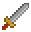# Input Mapper

InputMapper for mapping multiple input sources into actions! This can be useful for providing accessibility into your games and allowing users to map inputs to different game commands.

It also cleans up you input handling code by centralizing the source input and commands in one place.

``````const moveRight = (amount: number) => {
actor.vel.x = 100 * amount
}
const moveLeft = (amount: number) => {
actor.vel.x = -100 * amount
}
const moveUp = (amount: number) => {
actor.vel.y = -100 * amount
}
const moveDown = (amount: number) => {
actor.vel.y = 100 * amount
}
engine.inputMapper.on(
({ keyboard }) => (keyboard.isHeld(ex.Keys.ArrowRight) ? 1 : 0),
moveRight
)
engine.inputMapper.on(